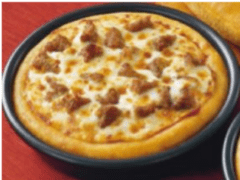### Submit a Resource

Mathalicious: Pizza Pi (HS)Which size pizza should you order?

Pizzas often come in three sizes: large, medium and personal. As you change the size of a pizza, how does the amount of cheesy, delicious inside compare to the amount of crust…and is it ever a good idea to buy the smallest size?

In this lesson, students use the formula for the area of a circle to calculate how much of a pizza is actually pizza, and how much is crust. They write linear and quadratic equations for these functions in terms of the radius (or diameter) of the pizza, and also express these areas as percents of the whole pie’s area. Finally, they talk about how they might use their newfound pizza knowledge to make the best decision when they want some pi(e).

*Requires paid membership to access lesson.

Algebra & Pre-Algebra, Geometry, Linear Equations, Quadratic Equations
High School

Mathalicious

Lesson Plan
PDF File